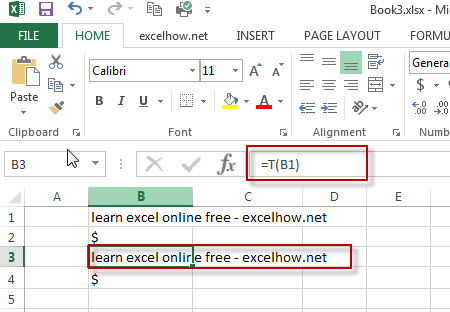# Excel T Function

This post will guide you how to use Excel T function with syntax and examples in Microsoft excel.

### Description

The Excel T function returns the text referred to by value

The T function is a build-in function in Microsoft Excel and it is categorized as a Text Function.

The T function is available in Excel 2016, Excel 2013, Excel 2010, Excel 2007, Excel 2003, Excel XP, Excel 2000, Excel 2011 for Mac.

### Syntax

The syntax of the T function is as below:

= T  (value)

Where the T function arguments are:
value -This is a required argument. The value you want to test.

Note:  If the value does not refer to a text string, the T function will return an empty text.

### Example

The below examples will show you how to use Excel T Text function to return the text string referred to by value.

#1 To return the text string value in B1, just using formula: =T(B1).